/NumPy 1.17

# The N-dimensional array (ndarray)

An ndarray is a (usually fixed-size) multidimensional container of items of the same type and size. The number of dimensions and items in an array is defined by its shape, which is a tuple of N non-negative integers that specify the sizes of each dimension. The type of items in the array is specified by a separate data-type object (dtype), one of which is associated with each ndarray.

As with other container objects in Python, the contents of an ndarray can be accessed and modified by indexing or slicing the array (using, for example, N integers), and via the methods and attributes of the ndarray.

Different ndarrays can share the same data, so that changes made in one ndarray may be visible in another. That is, an ndarray can be a “view” to another ndarray, and the data it is referring to is taken care of by the “base” ndarray. ndarrays can also be views to memory owned by Python strings or objects implementing the buffer or array interfaces.

#### Example

A 2-dimensional array of size 2 x 3, composed of 4-byte integer elements:

>>> x = np.array([[1, 2, 3], [4, 5, 6]], np.int32)
>>> type(x)
<type 'numpy.ndarray'>
>>> x.shape
(2, 3)
>>> x.dtype
dtype('int32')


The array can be indexed using Python container-like syntax:

>>> # The element of x in the *second* row, *third* column, namely, 6.
>>> x[1, 2]


For example slicing can produce views of the array:

>>> y = x[:,1]
>>> y
array([2, 5])
>>> y = 9 # this also changes the corresponding element in x
>>> y
array([9, 5])
>>> x
array([[1, 9, 3],
[4, 5, 6]])


## Constructing arrays

New arrays can be constructed using the routines detailed in Array creation routines, and also by using the low-level ndarray constructor:

 ndarray(shape[, dtype, buffer, offset, …]) An array object represents a multidimensional, homogeneous array of fixed-size items.

## Indexing arrays

Arrays can be indexed using an extended Python slicing syntax, array[selection]. Similar syntax is also used for accessing fields in a structured data type.

## Internal memory layout of an ndarray

An instance of class ndarray consists of a contiguous one-dimensional segment of computer memory (owned by the array, or by some other object), combined with an indexing scheme that maps N integers into the location of an item in the block. The ranges in which the indices can vary is specified by the shape of the array. How many bytes each item takes and how the bytes are interpreted is defined by the data-type object associated with the array.

A segment of memory is inherently 1-dimensional, and there are many different schemes for arranging the items of an N-dimensional array in a 1-dimensional block. NumPy is flexible, and ndarray objects can accommodate any strided indexing scheme. In a strided scheme, the N-dimensional index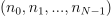corresponds to the offset (in bytes):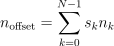from the beginning of the memory block associated with the array. Here,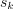are integers which specify the strides of the array. The column-major order (used, for example, in the Fortran language and in Matlab) and row-major order (used in C) schemes are just specific kinds of strided scheme, and correspond to memory that can be addressed by the strides: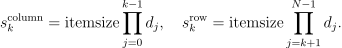where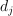= self.shape[j].

Both the C and Fortran orders are contiguous, i.e., single-segment, memory layouts, in which every part of the memory block can be accessed by some combination of the indices.

While a C-style and Fortran-style contiguous array, which has the corresponding flags set, can be addressed with the above strides, the actual strides may be different. This can happen in two cases:

1. If self.shape[k] == 1 then for any legal index index[k] == 0. This means that in the formula for the offset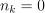and thus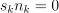and the value of= self.strides[k] is arbitrary.
2. If an array has no elements (self.size == 0) there is no legal index and the strides are never used. Any array with no elements may be considered C-style and Fortran-style contiguous.

Point 1. means that self and self.squeeze() always have the same contiguity and aligned flags value. This also means that even a high dimensional array could be C-style and Fortran-style contiguous at the same time.

An array is considered aligned if the memory offsets for all elements and the base offset itself is a multiple of self.itemsize. Understanding memory-alignment leads to better performance on most hardware.

Note

Points (1) and (2) are not yet applied by default. Beginning with NumPy 1.8.0, they are applied consistently only if the environment variable NPY_RELAXED_STRIDES_CHECKING=1 was defined when NumPy was built. Eventually this will become the default.

You can check whether this option was enabled when your NumPy was built by looking at the value of np.ones((10,1), order='C').flags.f_contiguous. If this is True, then your NumPy has relaxed strides checking enabled.

Warning

It does not generally hold that self.strides[-1] == self.itemsize for C-style contiguous arrays or self.strides == self.itemsize for Fortran-style contiguous arrays is true.

Data in new ndarrays is in the row-major (C) order, unless otherwise specified, but, for example, basic array slicing often produces views in a different scheme.

Note

Several algorithms in NumPy work on arbitrarily strided arrays. However, some algorithms require single-segment arrays. When an irregularly strided array is passed in to such algorithms, a copy is automatically made.

## Array attributes

Array attributes reflect information that is intrinsic to the array itself. Generally, accessing an array through its attributes allows you to get and sometimes set intrinsic properties of the array without creating a new array. The exposed attributes are the core parts of an array and only some of them can be reset meaningfully without creating a new array. Information on each attribute is given below.

### Memory layout

The following attributes contain information about the memory layout of the array:

 ndarray.flags Information about the memory layout of the array. ndarray.shape Tuple of array dimensions. ndarray.strides Tuple of bytes to step in each dimension when traversing an array. ndarray.ndim Number of array dimensions. ndarray.data Python buffer object pointing to the start of the array’s data. ndarray.size Number of elements in the array. ndarray.itemsize Length of one array element in bytes. ndarray.nbytes Total bytes consumed by the elements of the array. ndarray.base Base object if memory is from some other object.

### Data type

The data type object associated with the array can be found in the dtype attribute:

 ndarray.dtype Data-type of the array’s elements.

### Other attributes

 ndarray.T The transposed array. ndarray.real The real part of the array. ndarray.imag The imaginary part of the array. ndarray.flat A 1-D iterator over the array. ndarray.ctypes An object to simplify the interaction of the array with the ctypes module.

### Array interface

 __array_interface__ Python-side of the array interface __array_struct__ C-side of the array interface

### ctypes foreign function interface

 ndarray.ctypes An object to simplify the interaction of the array with the ctypes module.

## Array methods

An ndarray object has many methods which operate on or with the array in some fashion, typically returning an array result. These methods are briefly explained below. (Each method’s docstring has a more complete description.)

### Array conversion

 ndarray.item(*args) Copy an element of an array to a standard Python scalar and return it. ndarray.tolist() Return the array as an a.ndim-levels deep nested list of Python scalars. ndarray.itemset(*args) Insert scalar into an array (scalar is cast to array’s dtype, if possible) ndarray.tostring([order]) Construct Python bytes containing the raw data bytes in the array. ndarray.tobytes([order]) Construct Python bytes containing the raw data bytes in the array. ndarray.tofile(fid[, sep, format]) Write array to a file as text or binary (default). ndarray.dump(file) Dump a pickle of the array to the specified file. ndarray.dumps() Returns the pickle of the array as a string. ndarray.astype(dtype[, order, casting, …]) Copy of the array, cast to a specified type. ndarray.byteswap([inplace]) Swap the bytes of the array elements ndarray.copy([order]) Return a copy of the array. ndarray.view([dtype, type]) New view of array with the same data. ndarray.getfield(dtype[, offset]) Returns a field of the given array as a certain type. ndarray.setflags([write, align, uic]) Set array flags WRITEABLE, ALIGNED, (WRITEBACKIFCOPY and UPDATEIFCOPY), respectively. ndarray.fill(value) Fill the array with a scalar value.

### Shape manipulation

For reshape, resize, and transpose, the single tuple argument may be replaced with n integers which will be interpreted as an n-tuple.

 ndarray.reshape(shape[, order]) Returns an array containing the same data with a new shape. ndarray.resize(new_shape[, refcheck]) Change shape and size of array in-place. ndarray.transpose(*axes) Returns a view of the array with axes transposed. ndarray.swapaxes(axis1, axis2) Return a view of the array with axis1 and axis2 interchanged. ndarray.flatten([order]) Return a copy of the array collapsed into one dimension. ndarray.ravel([order]) Return a flattened array. ndarray.squeeze([axis]) Remove single-dimensional entries from the shape of a.

### Item selection and manipulation

For array methods that take an axis keyword, it defaults to None. If axis is None, then the array is treated as a 1-D array. Any other value for axis represents the dimension along which the operation should proceed.

 ndarray.take(indices[, axis, out, mode]) Return an array formed from the elements of a at the given indices. ndarray.put(indices, values[, mode]) Set a.flat[n] = values[n] for all n in indices. ndarray.repeat(repeats[, axis]) Repeat elements of an array. ndarray.choose(choices[, out, mode]) Use an index array to construct a new array from a set of choices. ndarray.sort([axis, kind, order]) Sort an array in-place. ndarray.argsort([axis, kind, order]) Returns the indices that would sort this array. ndarray.partition(kth[, axis, kind, order]) Rearranges the elements in the array in such a way that the value of the element in kth position is in the position it would be in a sorted array. ndarray.argpartition(kth[, axis, kind, order]) Returns the indices that would partition this array. ndarray.searchsorted(v[, side, sorter]) Find indices where elements of v should be inserted in a to maintain order. ndarray.nonzero() Return the indices of the elements that are non-zero. ndarray.compress(condition[, axis, out]) Return selected slices of this array along given axis. ndarray.diagonal([offset, axis1, axis2]) Return specified diagonals.

### Calculation

Many of these methods take an argument named axis. In such cases,

• If axis is None (the default), the array is treated as a 1-D array and the operation is performed over the entire array. This behavior is also the default if self is a 0-dimensional array or array scalar. (An array scalar is an instance of the types/classes float32, float64, etc., whereas a 0-dimensional array is an ndarray instance containing precisely one array scalar.)
• If axis is an integer, then the operation is done over the given axis (for each 1-D subarray that can be created along the given axis).

Example of the axis argument

A 3-dimensional array of size 3 x 3 x 3, summed over each of its three axes

>>> x
array([[[ 0,  1,  2],
[ 3,  4,  5],
[ 6,  7,  8]],
[[ 9, 10, 11],
[12, 13, 14],
[15, 16, 17]],
[[18, 19, 20],
[21, 22, 23],
[24, 25, 26]]])
>>> x.sum(axis=0)
array([[27, 30, 33],
[36, 39, 42],
[45, 48, 51]])
>>> # for sum, axis is the first keyword, so we may omit it,
>>> # specifying only its value
>>> x.sum(0), x.sum(1), x.sum(2)
(array([[27, 30, 33],
[36, 39, 42],
[45, 48, 51]]),
array([[ 9, 12, 15],
[36, 39, 42],
[63, 66, 69]]),
array([[ 3, 12, 21],
[30, 39, 48],
[57, 66, 75]]))


The parameter dtype specifies the data type over which a reduction operation (like summing) should take place. The default reduce data type is the same as the data type of self. To avoid overflow, it can be useful to perform the reduction using a larger data type.

For several methods, an optional out argument can also be provided and the result will be placed into the output array given. The out argument must be an ndarray and have the same number of elements. It can have a different data type in which case casting will be performed.

 ndarray.max([axis, out, keepdims, initial, …]) Return the maximum along a given axis. ndarray.argmax([axis, out]) Return indices of the maximum values along the given axis. ndarray.min([axis, out, keepdims, initial, …]) Return the minimum along a given axis. ndarray.argmin([axis, out]) Return indices of the minimum values along the given axis of a. ndarray.ptp([axis, out, keepdims]) Peak to peak (maximum - minimum) value along a given axis. ndarray.clip([min, max, out]) Return an array whose values are limited to [min, max]. ndarray.conj() Complex-conjugate all elements. ndarray.round([decimals, out]) Return a with each element rounded to the given number of decimals. ndarray.trace([offset, axis1, axis2, dtype, out]) Return the sum along diagonals of the array. ndarray.sum([axis, dtype, out, keepdims, …]) Return the sum of the array elements over the given axis. ndarray.cumsum([axis, dtype, out]) Return the cumulative sum of the elements along the given axis. ndarray.mean([axis, dtype, out, keepdims]) Returns the average of the array elements along given axis. ndarray.var([axis, dtype, out, ddof, keepdims]) Returns the variance of the array elements, along given axis. ndarray.std([axis, dtype, out, ddof, keepdims]) Returns the standard deviation of the array elements along given axis. ndarray.prod([axis, dtype, out, keepdims, …]) Return the product of the array elements over the given axis ndarray.cumprod([axis, dtype, out]) Return the cumulative product of the elements along the given axis. ndarray.all([axis, out, keepdims]) Returns True if all elements evaluate to True. ndarray.any([axis, out, keepdims]) Returns True if any of the elements of a evaluate to True.

## Arithmetic, matrix multiplication, and comparison operations

Arithmetic and comparison operations on ndarrays are defined as element-wise operations, and generally yield ndarray objects as results.

Each of the arithmetic operations (+, -, *, /, //, %, divmod(), ** or pow(), <<, >>, &, ^, |, ~) and the comparisons (==, <, >, <=, >=, !=) is equivalent to the corresponding universal function (or ufunc for short) in NumPy. For more information, see the section on Universal Functions.

Comparison operators:

 ndarray.__lt__(self, value, /) Return selfvalue. ndarray.__ge__(self, value, /) Return self>=value. ndarray.__eq__(self, value, /) Return self==value. ndarray.__ne__(self, value, /) Return self!=value.

Truth value of an array (bool):

 ndarray.__bool__(self, /) self != 0

Note

Truth-value testing of an array invokes ndarray.__bool__, which raises an error if the number of elements in the array is larger than 1, because the truth value of such arrays is ambiguous. Use .any() and .all() instead to be clear about what is meant in such cases. (If the number of elements is 0, the array evaluates to False.)

Unary operations:

 ndarray.__neg__(self, /) -self ndarray.__pos__(self, /) +self ndarray.__abs__(self) ndarray.__invert__(self, /) ~self

Arithmetic:

 ndarray.__add__(self, value, /) Return self+value. ndarray.__sub__(self, value, /) Return self-value. ndarray.__mul__(self, value, /) Return self*value. ndarray.__truediv__(self, value, /) Return self/value. ndarray.__floordiv__(self, value, /) Return self//value. ndarray.__mod__(self, value, /) Return self%value. ndarray.__divmod__(self, value, /) Return divmod(self, value). ndarray.__pow__(self, value[, mod]) Return pow(self, value, mod). ndarray.__lshift__(self, value, /) Return self<>value. ndarray.__and__(self, value, /) Return self&value. ndarray.__or__(self, value, /) Return self|value. ndarray.__xor__(self, value, /) Return self^value.

Note

• Any third argument to pow is silently ignored, as the underlying ufunc takes only two arguments.
• The three division operators are all defined; div is active by default, truediv is active when __future__ division is in effect.
• Because ndarray is a built-in type (written in C), the __r{op}__ special methods are not directly defined.
• The functions called to implement many arithmetic special methods for arrays can be modified using __array_ufunc__.

Arithmetic, in-place:

 ndarray.__iadd__(self, value, /) Return self+=value. ndarray.__isub__(self, value, /) Return self-=value. ndarray.__imul__(self, value, /) Return self*=value. ndarray.__itruediv__(self, value, /) Return self/=value. ndarray.__ifloordiv__(self, value, /) Return self//=value. ndarray.__imod__(self, value, /) Return self%=value. ndarray.__ipow__(self, value, /) Return self**=value. ndarray.__ilshift__(self, value, /) Return self<<=value. ndarray.__irshift__(self, value, /) Return self>>=value. ndarray.__iand__(self, value, /) Return self&=value. ndarray.__ior__(self, value, /) Return self|=value. ndarray.__ixor__(self, value, /) Return self^=value.

Warning

In place operations will perform the calculation using the precision decided by the data type of the two operands, but will silently downcast the result (if necessary) so it can fit back into the array. Therefore, for mixed precision calculations, A {op}= B can be different than A = A {op} B. For example, suppose a = ones((3,3)). Then, a += 3j is different than a = a + 3j: while they both perform the same computation, a += 3 casts the result to fit back in a, whereas a = a + 3j re-binds the name a to the result.

Matrix Multiplication:

 ndarray.__matmul__(self, value, /) Return self@value.

Note

Matrix operators @ and @= were introduced in Python 3.5 following PEP465. NumPy 1.10.0 has a preliminary implementation of @ for testing purposes. Further documentation can be found in the matmul documentation.

## Special methods

For standard library functions:

 ndarray.__copy__() Used if copy.copy is called on an array. ndarray.__deepcopy__() Used if copy.deepcopy is called on an array. ndarray.__reduce__() For pickling. ndarray.__setstate__(state, /) For unpickling.

Basic customization:

 ndarray.__new__(\*args, \*\*kwargs) Create and return a new object. ndarray.__array__() Returns either a new reference to self if dtype is not given or a new array of provided data type if dtype is different from the current dtype of the array. ndarray.__array_wrap__()

Container customization: (see Indexing)

 ndarray.__len__(self, /) Return len(self). ndarray.__getitem__(self, key, /) Return self[key]. ndarray.__setitem__(self, key, value, /) Set self[key] to value. ndarray.__contains__(self, key, /) Return key in self.

Conversion; the operations int, float and complex. . They work only on arrays that have one element in them and return the appropriate scalar.

 ndarray.__int__(self) ndarray.__float__(self) ndarray.__complex__()

String representations:

 ndarray.__str__(self, /) Return str(self). ndarray.__repr__(self, /) Return repr(self).